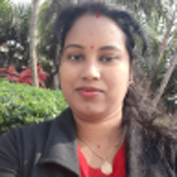# Differential Equations and Linear AlgebraTeacher

##### Mrs. Saubhagyalaxmi SinghCategory

### Course Attendees

Still no participant

Still no reviews

# Code(Credit) :CUTM1001 (2-0-1)

## Course Objectives

• Introduce students to how to solve linear Differential Equations with different methods.
• To solve the system of linear equations appearing in the problems of electrical engineering, mechanical engineering etc.
• To use Eigen values and Eigen vectors in Control theory, vibration analysis, electric circuits, advanced dynamics problems.
• Introduce students how to solve first order and second order differential equations

## Learning Outcomes

Upon successful completion of this course, students will be able to:
• Understand the importance of linear functions in mathematics.
• Solve systems of linear equations using Gauss- elimination to reduce to echelon form.
• Learn fundamental concepts of ODE theories and where and how such equations arise in applications to scientific and engineering problems.
• Be competent in solving linear/non-linear 1st & higher order ODEs using analytical methods to obtain their exact solutions

## Course Syllabus

Module-I:
First order linear differential equations and its applications

Project-1: Some applications of differential equations in RL-RC electrical circuit problems

Module-II:
Second order linear homogeneous differential equations (Real roots, Real equal roots, Complex conjugate roots) and its applications.

Project-2: RLC Circuit, Pendulum

Module-III:
Second order linear non-homogeneous differential equations, Finding particular integral consisting of exponential, trigonometric (Sine, cosine) using inverse operator method

Project-3: Simple mass-spring system, Damped vibration system

Module-IV:
Basic concepts of a matrices, solution of linear system of equations by Gauss elimination method, linearly independent and dependent of a vectors, rank of a matrix.

Project 4: Report on finding the traffic flow in the net of one-way streets

Module-V:
Determinants and Cramer’s Rule, Fundamental theorem of linear system of equations.

Module-VI:
Eigenvalues and Eigen vectors of a matrix.

Project 5: (i)Find the limit states of the Markov process model.
(ii)Find the growth rate in the Leslie model

Module-VII:
Symmetric, Skew-Symmetric , Orthogonal Matrices and Properties

Project 6: To make a report to show that the product of two orthogonal matrices is orthogonal, and so is the inverse of an orthogonal matrix. What does this mean in terms of rotations?

Text Books:
1. Advanced engineering mathematics by Erwin Kreyszig, 8th edition
Chapter-6 (6.1-6.6), Chapter-7 (7.1,7.2)
2. Higher Engineering by B.V. Ramana
Chapter-8(8.1,8.2,8.9,8.10,8.21), Chapter-9 (9.2,9.3,9.5)

Reference Books:
1. J. Sinha Roy and S. Padhy, A Course of Ordinary and Partial Differential Equations, Kalyani Publishers, New Delhi.
2. G.B. Thomas, M.D. Weir, J.R. Hass, Thomas’ Calculus, Pearson Publication.
3. R.G. Bartle, D.R. Sherbert, Introduction to Real Analysis, Wiley Publication

## Session 1

Overview of differential equations
Overview of differential equations

## Session 2

First order linear differential equations
First order linear differential equations

## Session 3

Applications of First order linear differential equations( Kirchhoff's law)
Kirchhoff's law

## Session 4 & 5

Project-1 (2 hrs)

RL-RC electrical circuit problems
RL-RC electrical circuit problems

## Session 6

Second order linear homogeneous differential equations
Second order linear homogeneous differential equations

## Session 7

Solution of Second order linear homogeneous differential equations
Second order linear homogeneous differential equations

## Session 8

Applications of Second-Order Differential Equations
Applications of Second-Order Differential Equations

## Session 9 & 10

Project-2 (2 hrs)

RLC Circuit
RLC Circuit

## Session 11

Second order linear non-homogeneous differential equations
Second order linear non-homogeneous differential equations

## Session 13

Finding particular integral consisting of trigonometric (Sine, cosine) using inverse operator method
https://www.youtube.com/watch?v=r6mkGRLpm_w

## Session 14 & 15

Project-3 (2 hrs)

Simple mass-spring system
https://www.youtube.com/watch?v=FJBPNJR2QJU

## Session 17

Linear system of equations by Gauss elimination method
Linear algebra
https://www.youtube.com/watch?v=2j5Ic2V7wq4

## Session 19 & 20

Project-4 (Flip Class) (2 hrs)
Report on finding the traffic flow in the net of one-way streets

## Session 21

Determinants of a matrix, Cramer’s Rule
Determinant
Linear algebra
https://www.youtube.com/watch?v=Ip3X9LOh2dk

## Session 23

Eigenvalues and Eigen Vectors
https://www.youtube.com/watch?v=PFDu9oVAE-g

## Session 25

Some application problems of Eigenvalues and Eigen Vectors
Eigen values and eigen functions
https://www.youtube.com/watch?v=R13Cwgmpuxc

## Session 26 & 27

Project-5 (Flip Class) (2hrs)
(i)Find the limit states of the Markov process model.
(ii)Find the growth rate in the Leslie model

## Session 28

Symmetric, Skew-Symmetric and Orthogonal Matrices
Symmetric matrix
https://www.youtube.com/watch?v=v5abfTlztTc

## Session 29

Properties of Symmetric, Skew-Symmetric and Orthogonal Matrices
https://www.youtube.com/watch?v=v5abfTlztTc

## Session 30

Problems of Symmetric, Skew-Symmetric and Orthogonal Matrices
https://www.youtube.com/watch?v=v5abfTlztTc

symmetric matrix

## Session 31 & 32

Project-6 (Flip class) (2 hrs)

To make a report to show that the product of two orthogonal matrices is orthogonal, and so is the inverse of an orthogonal matrix. What does this mean in terms of rotations?

## Recent Comments

### Our Main Teachers### Mrs. Saubhagyalaxmi Singh

##### Assistant Professor in School of Applied Sciences, Department of Mathematics
VIEW PROFILE

Mrs. Saubhagyalaxmi Singh works as an Assistant Professor, Department of Mathematics, Centurion University of Technology and Management. She has over 6 years of teaching experience with exposure to Utkal University, Bhubaneswar, Department of Mathematics. She has published 6 papers communicated to different International Journals. She has guided 7 M.Sc. projects in her area. She has […]